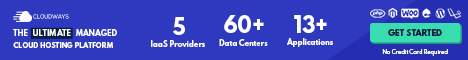# Economic Growth

## What Is Economic Growth?

Economic growth is simply the rise in the economy's capacity to produce goods and services.

Economic growth, in other words, refers to the ability of the economy to produce increasing quantities of goods and services.

An economy Opens in new window can only be said to be growing if value added across all productive activities taken together is positive.

That is, an economy grows only if the value of output in total is greater than the value of all of the resources that were used up during production.

One of the greatest of all economic fallacies is the belief that it is the using up of resources that creates growth, which is the concept behind Keynesian economic theory Opens in new window.

But it is not using resources in ‘any which way’ that is value adding.

Only if there were more value at the end of the process than existed at the beginning could it be said that genuine value-adding activity has occurred and the economy has grown.

### Calculating the Economic Growth Rate

To measure the rate of economic growth we usually do so by calculating the rate of change in real GDP Opens in new window from one year to the next.

Therefore the economic growth rate is measured as the rate of change of real GDP from one year to the next.

Australia has, over time, usually experienced positive economic growth rates, which is essential if standards of living are to rise and employment is to grow, and to ensure that the country can provide for a growing population.

Real GDP Opens in new window for Australia in the financial year 2011/12 was \$1 451 824 million and in 2012/13 it was \$1 493 171 million.

From these figures we can calculate the rate of economic growth between those two years as follows:

We can now say that between 2011/12 and 2012/13 the real value of goods and services in Australia grew by 2.8 percent.### The GDP Deflator

Economists and policy-makers are interested not just in the level of total production, as measured by real GDP, but also in the price level.

The price level measures the average prices of goods and services in the economy.

One of the goals of economic policy is a stable price level. We can use values for nominal GDP and real GDP Opens in new window to calculate a measure of the price level, called the GDP deflator.

The GDP deflator is a measure of the price level, calculated by dividing nominal GDP by real GDP and multiplying by 100.

We can calculate the GDP deflator by using this formula:

To see why the GDP deflator is a measure of the price level, think about what would happen if prices of goods and services rose while production remained the same.

In that case, nominal GDP would increase but real GDP would remain constant, so the GDP deflator would increase.

In reality, both prices and production usually increase each year, but the more prices increase relative to the increase in production, the more nominal GDP increases relative to real GDP and the higher the value for the GDP deflator.

Increases in the GDP deflator allow economists and policy-makers to track increases in the price level over time.

2011/122012/13
Nominal GDP\$1 474 291 million\$1 510 917 million
Real GDP\$1 451 824 million\$1 493 171 million

We can use the information from the table to calculate values for the GDP price deflator for 2011/12 and 2012/13.

From these values for the deflator, we can calculate that the price level barely changed between 2011/12 and 2012/13—in fact, it fell very slightly, by 0.3 percent:

This is unusual as in most years the price level rises. However, between 2011/12 and 2012/13 prices for commodity exports fell (by an average of just over 3 percent) along with falling prices of some other tradable goods.

The combination of price falls in some areas of the economy together with price levels when measured using the GDP deflator.